Next: References Up: A COMPLETE TREATMENT OF Previous: Radial Functions

# Derivation of the Normal Modes

We would like to describe the spatial and angular dependence of the normal modes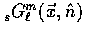in a coordinate-free way by constructing them out of covariant derivatives of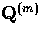contracted with some orthonormal basis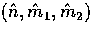.The lowest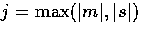modes can be written as [3,4],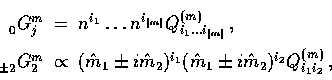and satisfy (Appendix B),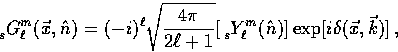(55)
with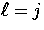. We demand that the higher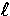-modes also do so, to maintain the division of spin and orbital angular momentum defined in flat space .

We begin the construction by choosing some arbitrary point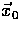,and using a spherical coordinate system around it,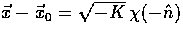.Now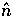defines both the intrinsic angular coordinate system and the angular coordinates for the spatial location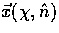.This reduction in the dimension of the space is sufficient since the end goal is to derive how the intrinsic and orbital angular dependence in the same directionadds. In physical terms, only those photons directed toward the observer can contribute to the local angular dependence there. First expand the lowest mode in spin-spherical harmonics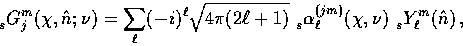(56)
where recall that the dimensionless wavenumber is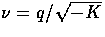.We obtain the explicit expressions for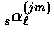and their recursion relations in Appendix B by simple comparison between equations (C1) and (C3). At the origin they satisfy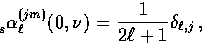(57)
which both fixes the normalization of the modes and manifestly obeys Eq. (C2). As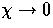only the local angular dependence remains, as expressed in the Kronecker delta of Eq. (C4). Because the spatial variation of the normal mode Q(m) across a shell at fixed radius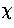must be added to the local dependence, even a mode of fixed j has a sum over allin its angular dependence which contributes at any other point.

This generation of higherstructure asincreases suggests that we can use the radial structure of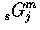to generate the highermodes. From the radial recursion relation forEq. (B9), let us make the ansatz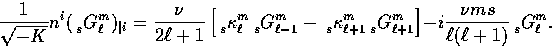(58)
That this series generates modes with the desired properties can be shown by returning to the spherical coordinate system. By explicit substitution of the radial form forof Eq. (C3) and by noting that in this coordinate system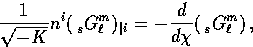(59)
we obtain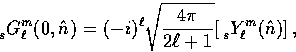(60)
(up to a phase factor) as desired. Since we have shown this for an arbitrary point, it is clear that Eq. (C2) holds in general. Note that this construction requires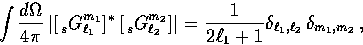(61)
for all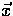, as in the flat case of Eq. (24), and defines our normalization convention.Next: References Up: A COMPLETE TREATMENT OF Previous: Radial Functions
Wayne Hu
9/9/1997